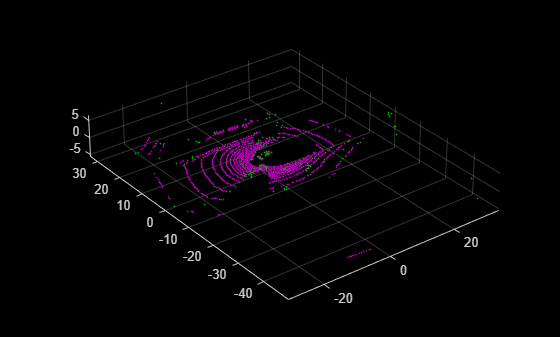# show

Visualize LOAM map

Since R2022b

## Syntax

``show(loamMap)``
``show(loamMap,Name=Value)``
``ax = show(___)``

## Description

example

````show(loamMap)` displays the edge and surface points contained in the input lidar odometry and mapping (LOAM) map. The function displays surface points in magenta and edge points in green.```
````show(loamMap,Name=Value)` specifies options using one or more name-value argument. For example, `MarkerSize=5` sets the diameter size of the marker to `5` points.```
````ax = show(___)` returns the plot axes using any combination of input arguments from previous syntaxes.```

## Examples

collapse all

Create a LOAM map to store LOAM feature points.

```voxelSize = 0.5; loamMap = pcmaploam(voxelSize);```

Load point cloud data into the workspace.

`ld = load("drivingLidarPoints.mat");`

Detect LOAM feature points.

`points = detectLOAMFeatures(ld.ptCloud);`

Add the LOAM points to the map.

```absPose = rigidtform3d; addPoints(loamMap,points,absPose)```

Visualize the points in the LOAM map.

`show(loamMap)`## Input Arguments

collapse all

LOAM map, specified as a `pcmaploam` object.

### Name-Value Arguments

Specify optional pairs of arguments as `Name1=Value1,...,NameN=ValueN`, where `Name` is the argument name and `Value` is the corresponding value. Name-value arguments must appear after other arguments, but the order of the pairs does not matter.

Example: `show(loamMap,MarkerSize=5)` sets the diameter size of the marker to `5` points.

Diameter of each marker, specified as a positive scalar. The value specifies the approximate diameter of the markers used for each LOAM point in the display. MATLAB® graphics defines the unit as points.

Axes on which to display the visualization, specified as an `Axes` object. To create an `Axes` object, use the `axes` function. To display the visualization in a new figure, leave `Parent` unspecified.

## Output Arguments

collapse all

Plot axes, returned as an `axes` graphics object. You can set the default center of rotation for the viewer as around the axes center or a point. Set the default behavior from the Computer Vision Toolbox Preferences.

## Version History

Introduced in R2022b